# 1617-257/TUT-R-7

Suppose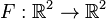$F : \mathbb{R}^2 \to \mathbb{R}^2$ is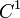$C^1$ with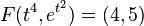$F(t^4, e^{t^2}) = (4,5)$ for all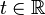$t \in \mathbb{R}$. Prove that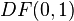$DF(0,1)$ is not invertible.
Let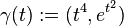$\gamma(t) := (t^4, e^{t^2})$. At the end of the tutorial, a couple of students pointed out that it's also true that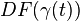$DF(\gamma(t))$ is not invertible for any$t \in \mathbb{R}$. It's easy to check that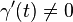$\gamma'(t) \neq 0$ when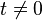$t \neq 0$ and that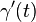$\gamma'(t)$ is in the kernel of$DF(\gamma(t))$.
It takes some more care to prove what the problem is asking because one can't immediately deduce that$DF(\gamma(t))$ has non-trivial kernel with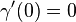$\gamma'(0) = 0$. A key observation here is that since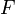$F$ is$C^1$, invertibility of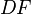$DF$ at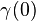$\gamma(0)$ implies invertibility of$DF$ at a neighborhood of$\gamma(0)$.